Abstract: 数字图像处理：第47天
Keywords: 边缘检测,LoG算子

# 灰度图像-图像分割 Marr-Hildreth算子（LoG算子）

#LoG原理
LoG最底层的原理是二阶微分算子，也就是对原始图像求二次微分的边缘定位算法，前面的边缘模型中可以得知，当使用二阶微分算子的时候，其对边缘的响应是一个零交叉，而且能够判断出高灰度方向，但二阶微分对噪声的敏感度过高，需要平滑预处理。
Marr和Hildreth【Marr和Hildreth，1980】证明了以下两个观点：

（1）灰度变化与图像尺寸没有关系，因此检测需要不同尺度的算子
（2）灰度的突然变化会在一阶导数中引起波峰和波谷，或者二阶导数中一起零交叉

## 数学原理

LoG算子： $\nabla^2G$

$G(x,y)=e^{-\frac{x^2+y^2}{2\delta^2}}$

$\nabla^2G(x,y)=\frac{\partial^2G(x,y)}{\partial x^2}+\frac{\partial^2G(x,y)}{\partial y^2}=[\frac{x^2}{\delta^4}-\frac{1}{\delta^2}]e^{-\frac{x^2+y^2}{2\delta^2}}$

$\nabla^2G(x,y)=[\frac{x^2+y^2-2\delta^2}{\delta^4}]e^{-\frac{x^2+y^2}{2\delta^2}}$

LoG零交叉出现在$x^2+y^2=2\delta^2$处LoG函数形状如下图，也被叫做墨西哥草帽算子。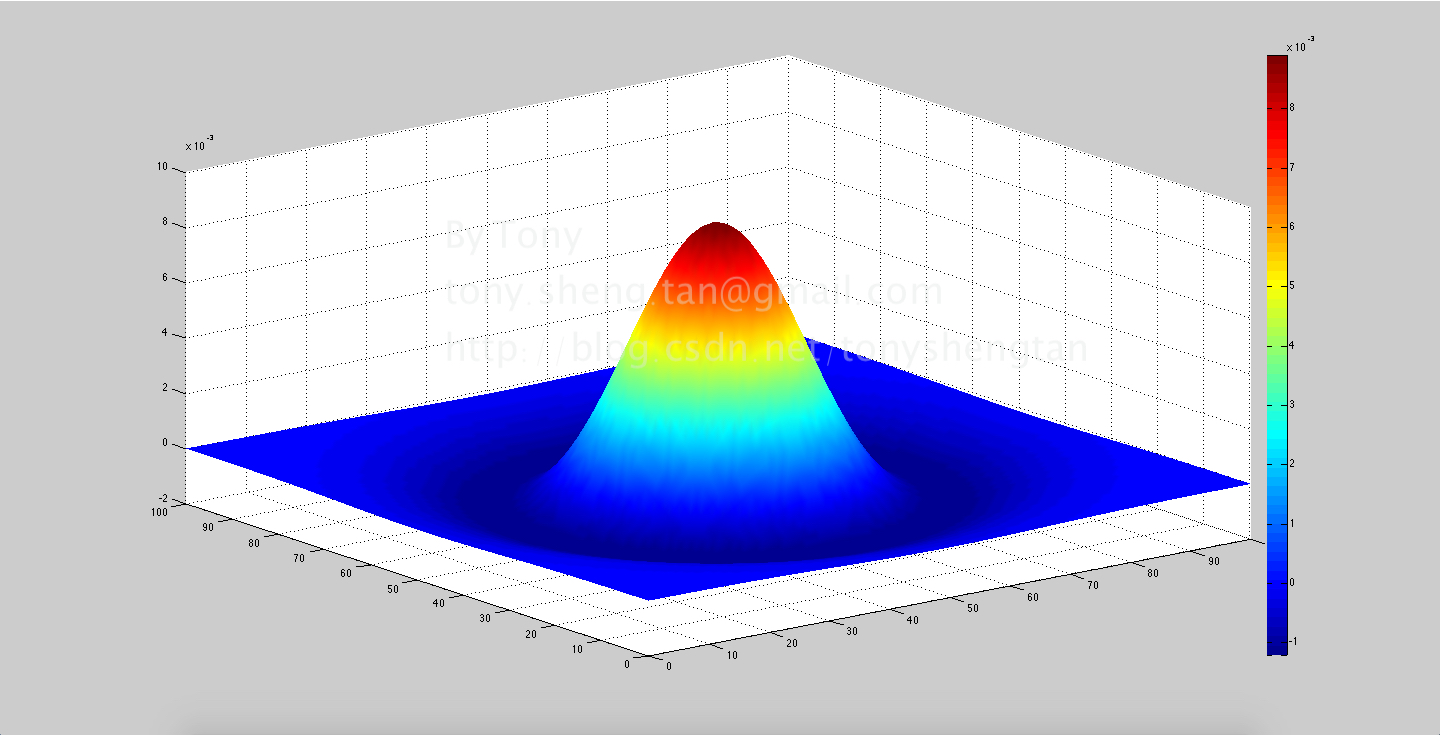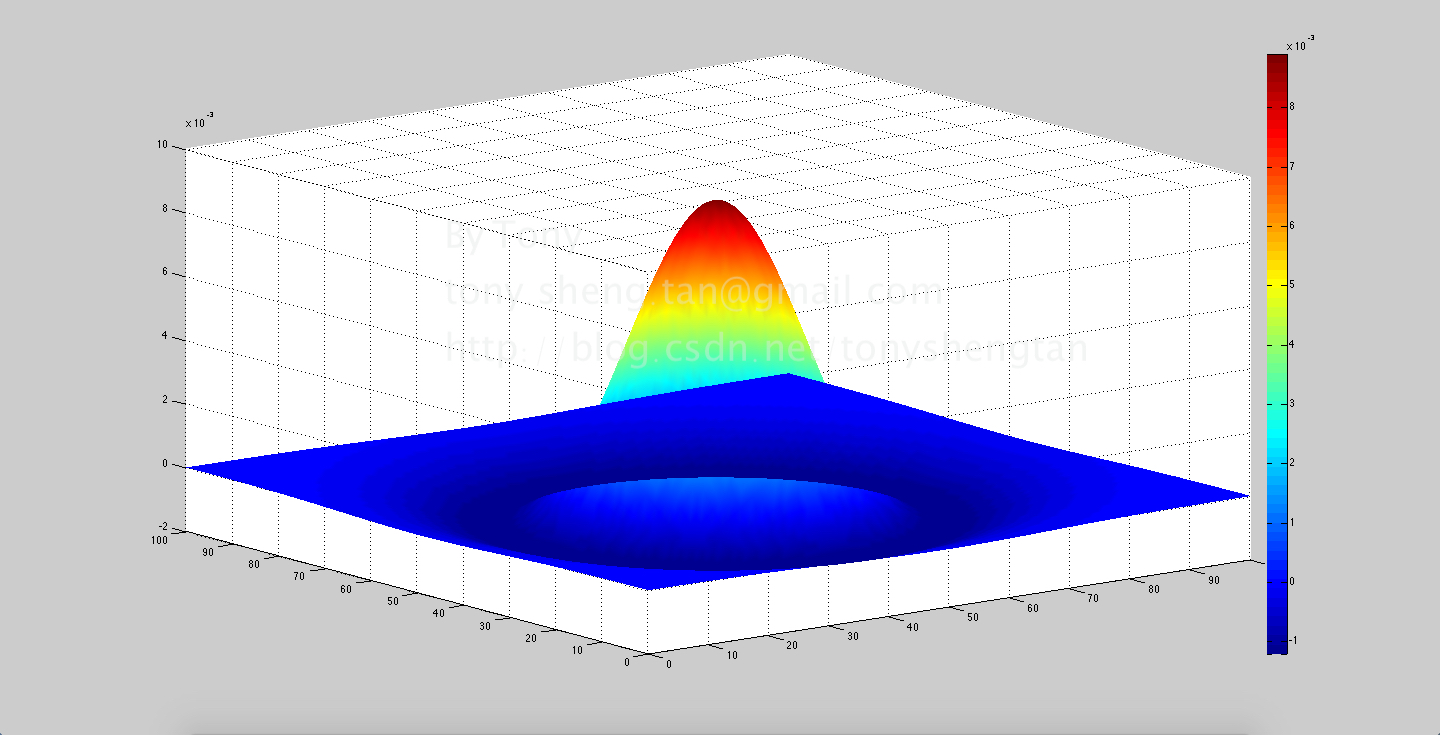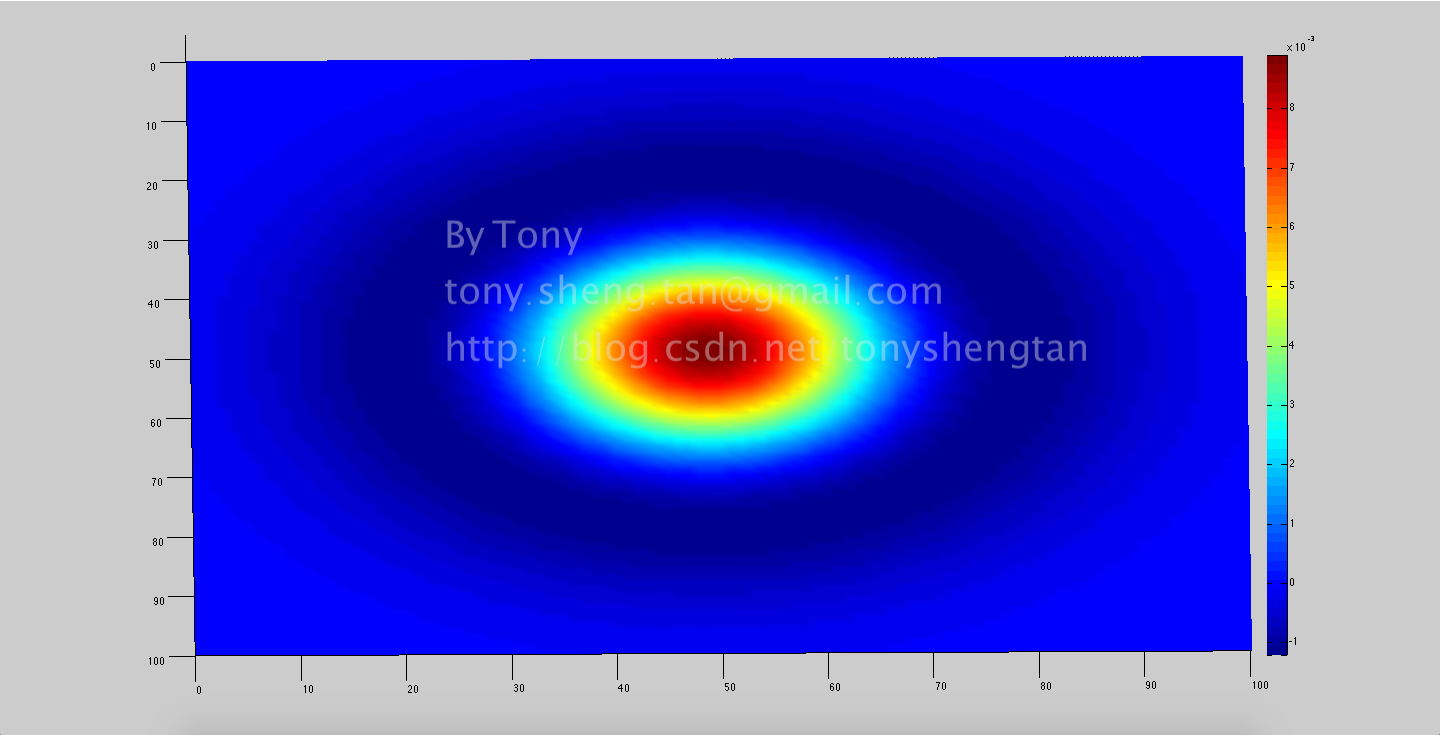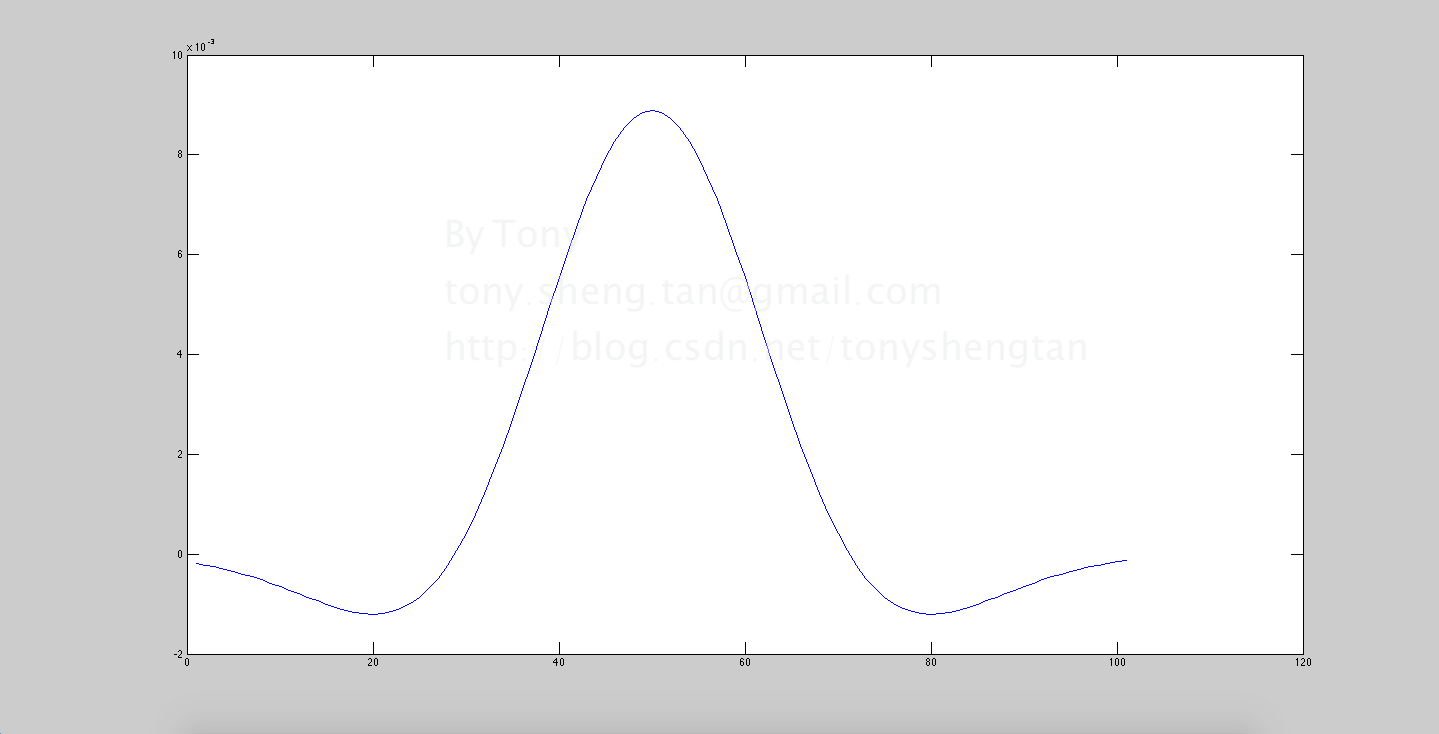## 模板性质

• 性质1，该模板对平坦区域应该无响应，所以算子内系数和应该为0，使用公式计算出来的结果不为零，需要整体上下平移模板
• 性质2，LoG可以使用laplace算子和高斯模板进行卷积后求得，其等效于使用LoG大小和标准差的高斯平滑后得到laplace结果
• 性质3，得到的结果要检测零交叉来定位边缘，因为噪声等原因，这里进行判断时可以使用阈值，也就是当出现零交叉的时候还要判断下正负值的差的绝对值是否满足阈值要求。
• 性质4，LoG模板的大小和标准差的选择关系，遵循高斯分布的$3\delta$原则，也就是当位置超过均值正负$3\delta$以外的值很小，也就是说，LoG模板应该选择大于$6\delta$的最小奇数作为模板大小，过大效果不会有提高反而增加计算量，过小会造成截断，无法得到正确结果。

## 操作结果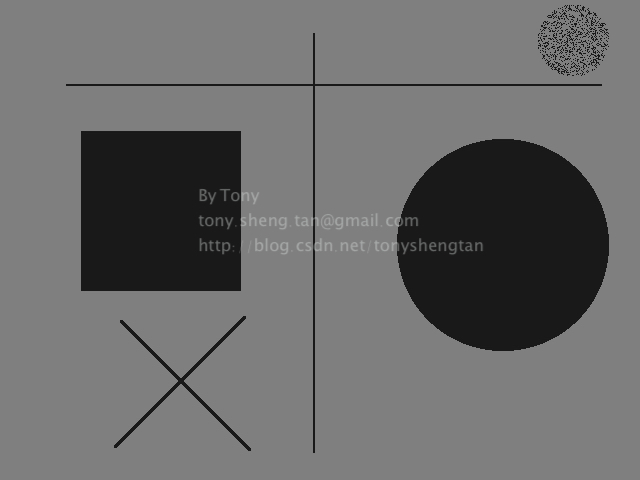LoG结果：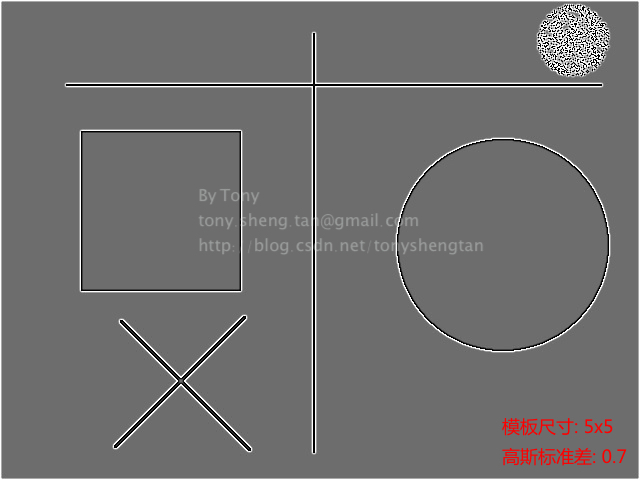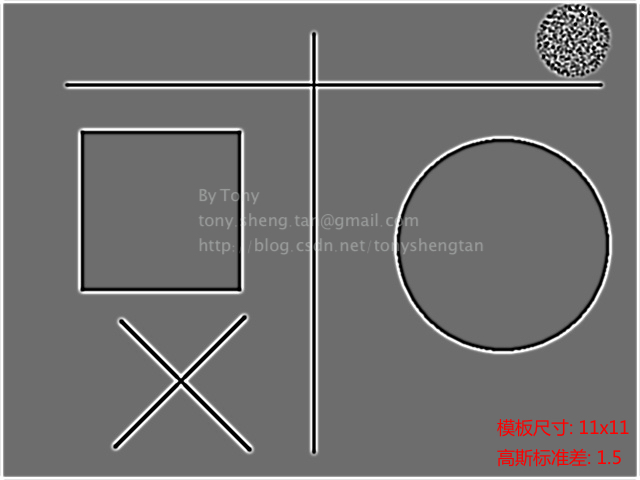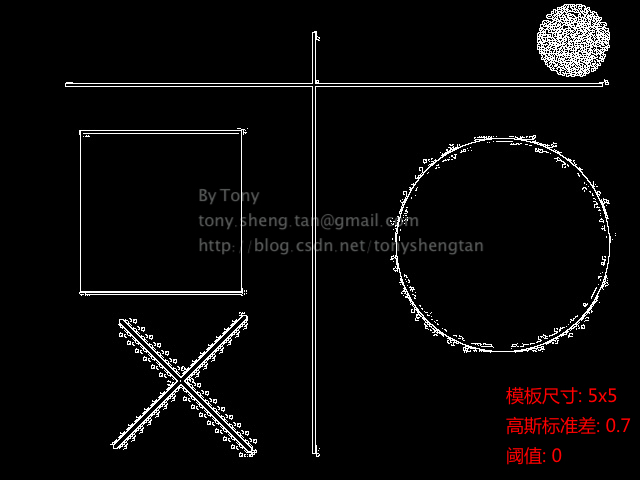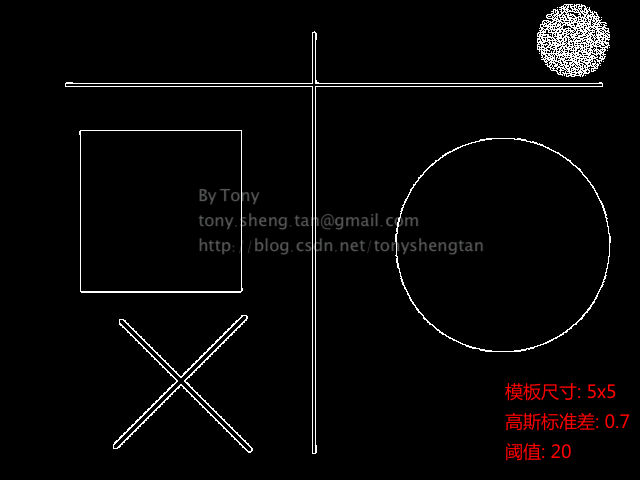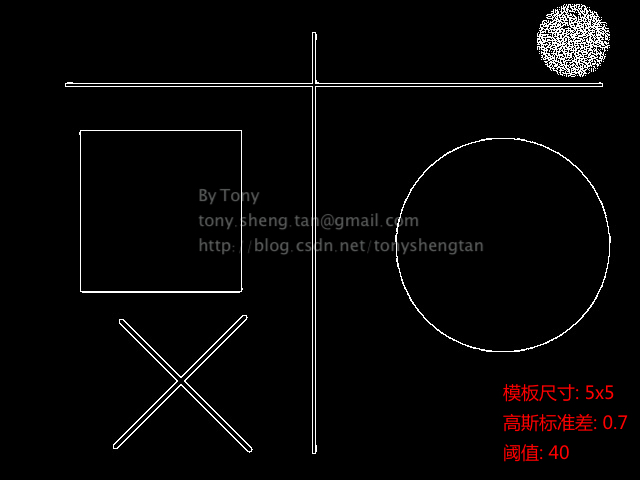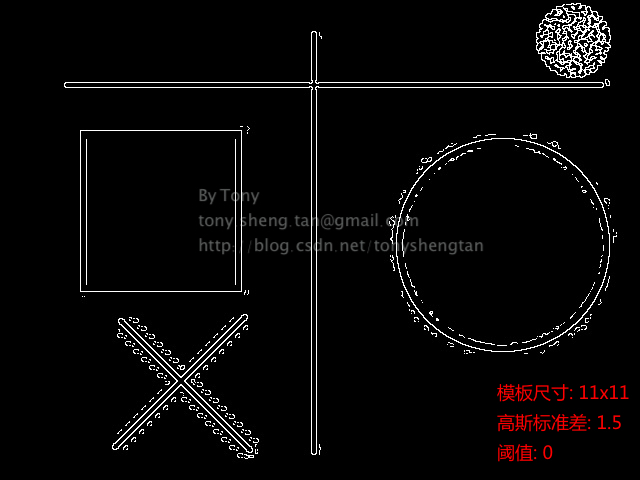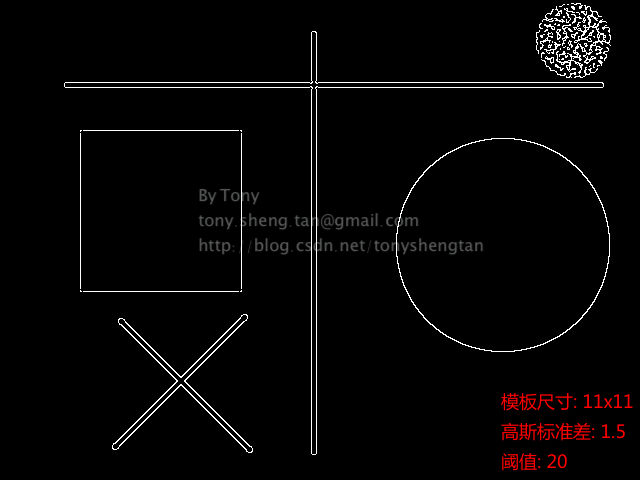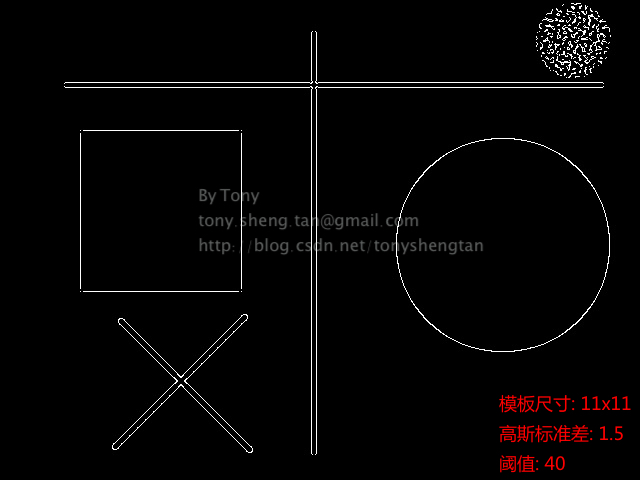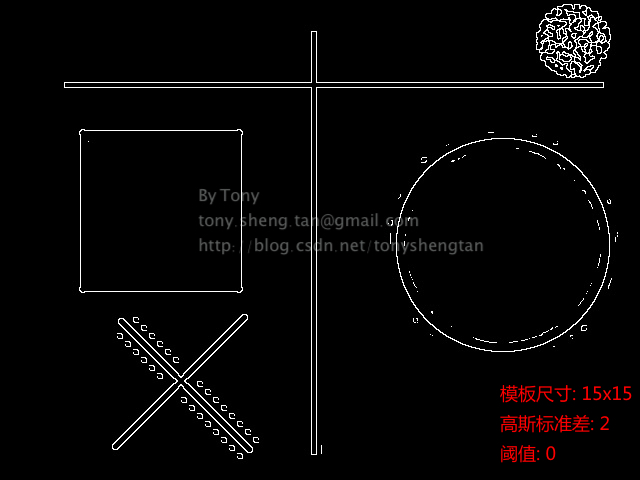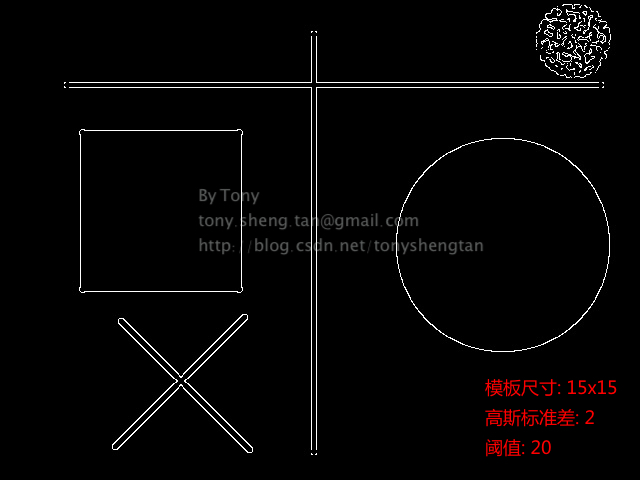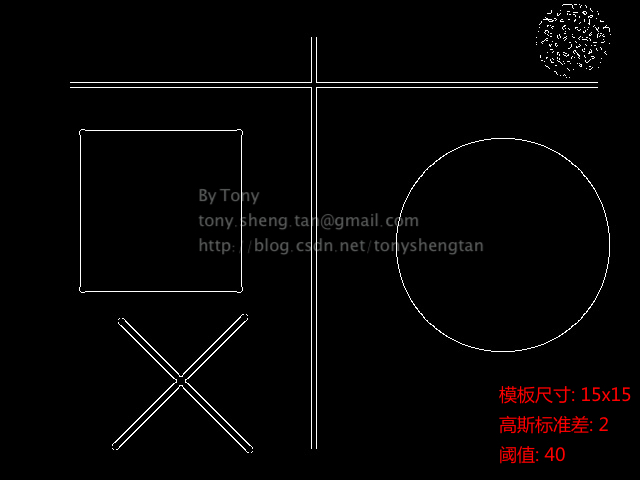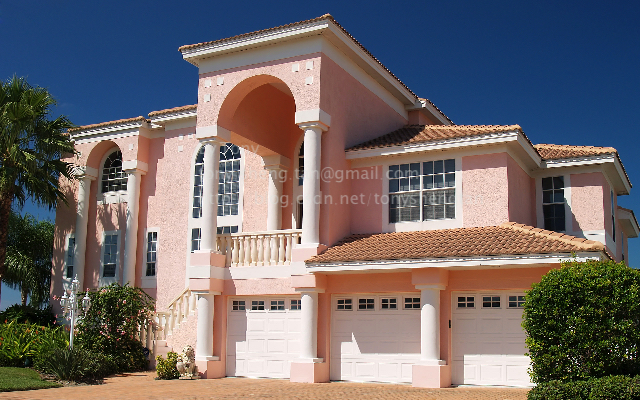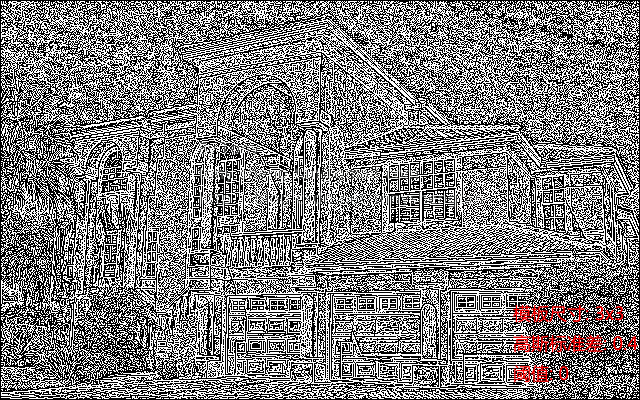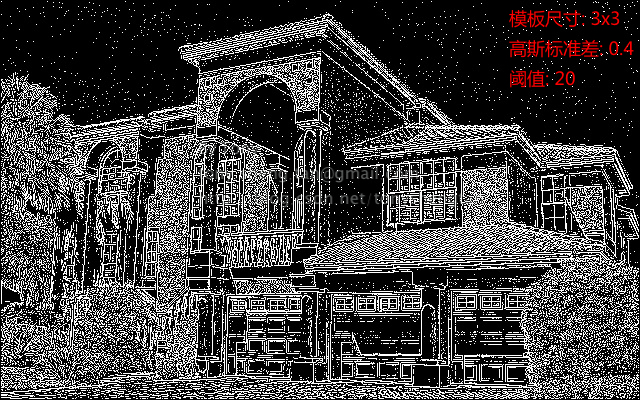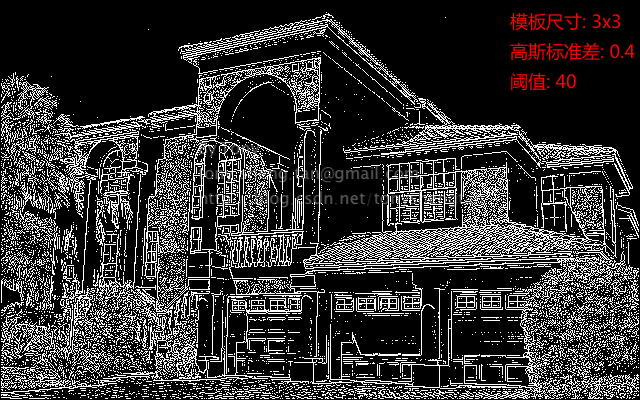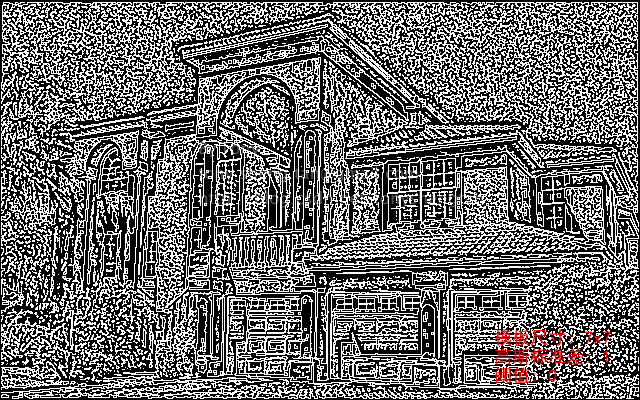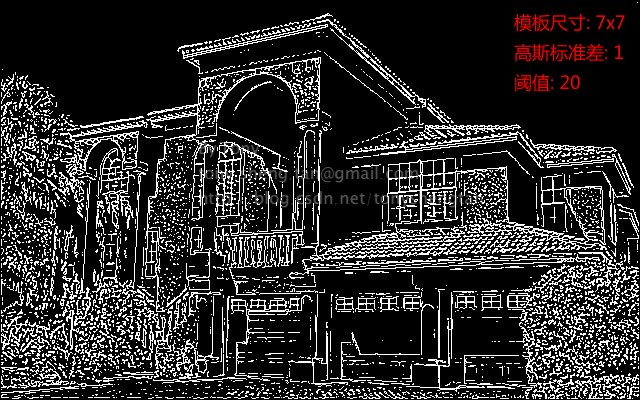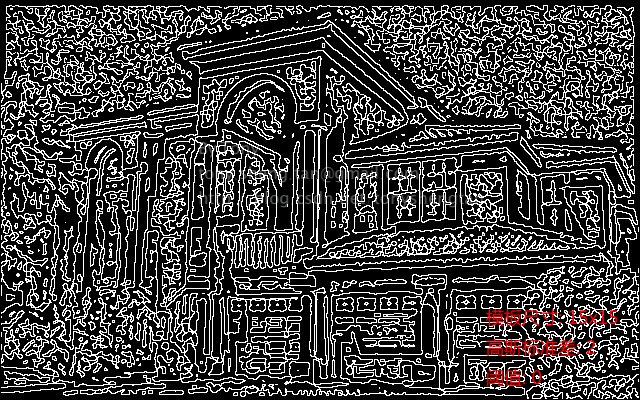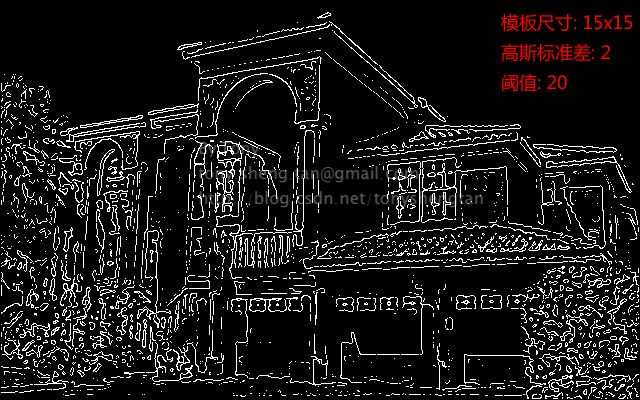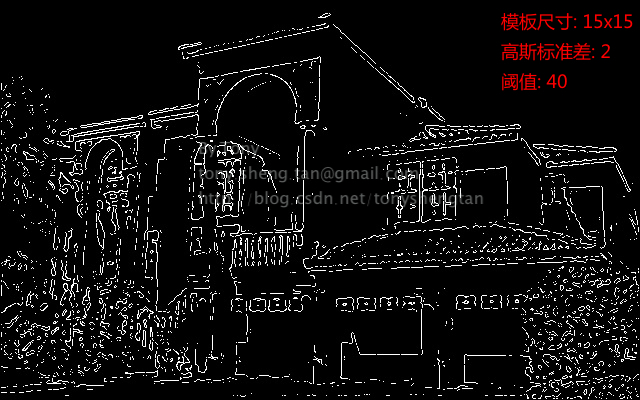0%